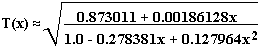# Round Wire Inductance Calculator

Calculates the Inductance of a single round wire of common conducting materials using the formula and data referenced below.

 Select the conducting material: Aluminium Copper Gold Nickel Silver Relative Permeability (μr): Enter the required frequency: MHz Conductance (σ): x 107 S/m Enter the length of the conductor: cm x: Enter the diameter of the conductor: mm T(x): Inductance: nH

The original formula below has been changed to use diameter rather than radius. Also the units have been changed from µH to nH and the diameter from cm to mm to make the calculator more practical.

This calculator uses JavaScript and will function in most modern browsers. For more information see About our calculators

From Transmission Line Design Handbook by Brian C Wadell, Artech House 1991 page 382 paragraph 6.2.1.1

The self inductance of a straight wire at high frequency is:Where d is the diameter in cm

l is the Length in cm

f is the Frequency in Hz

The formula originates from Inductance Calculations by F W Grover, Van Nostrand, Princeton, NJ, 1947, Reprinted by Dover Publications, NY, 1962. Two more formulas are given by Brian Wadell for calculating T(x) which are "accurate to a few percent over the range 0.0 ≤ x ≤ 100.0".Wherer is the radius of the conductor in cm

μ is the absolute magnetic permeability of the conductor

The absolute magnetic permeability (μ) = μo x μr

μo  = 4π x 10-7 H/m

The values for μr and σ are from Transmission Line Design Handbook by Brian C Wadell, Artech House 1991 Table 9.3.2 page 446.

This calculator is provided free by Chemandy Electronics in order to promote the FLEXI-BOX